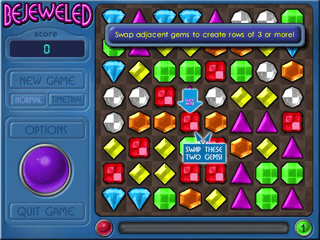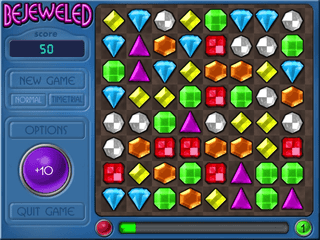top of page
Search
•Joseph Woolf

# Bejeweled 1 AI (Part 2): Enabling AI to Make Moves

Updated: Dec 18, 2021

In my previous post, I was able to get our code to get past the loading screen and get the board information.  However, a program that can only grab the board without acting on the information does no good.  In this post, we’ll be adding the basic mechanisms for our AI to act on the board information.  Please note that more intelligent behavior won’t be added in this post.

### Analyze Potential Moves

Now that our program can return us the board information, we need to figure out where our AI can make a move.

We represented our board as an 8×8 array.  Using that information, we can come with a diagram of the following valid moves:A diagram on how a computer can see valid moves.

From the diagram, there are a maximum of six valid moves that you can make if the same pieces are adjacent to each other.  In the case that the same pieces are a space apart, only two moves can be made.  These moves are the basis for more advanced matches, like 4-piece and T-shape.

### Code Base

Now that we have defined a valid move, let’s write up the code to detect valid moves.  I split up horizontal and vertical search into different methods for better readability.  A valid move is represented by the text “({x},{y}){d}”

For the case of horizontal search:

```def horizontalSearch(self, board):
moves = []
for y in range(8):
occur = 0
curColor = ""
for x in range(8):
if board[y][x] != curColor:
curColor = board[y][x]
if x+2 < 8 and board[y][x+2] == curColor:
if y-1 >= 0 and board[y-1][x+1] == curColor:
moves.append("({},{}){}".format(x+1,y, "U"))
if y+1 < 8 and board[y+1][x+1] == curColor:
moves.append("({},{}){}".format(x+1,y, "D"))
else:
if x-3 >= 0 and board[y][x-3] == curColor:
moves.append("({},{}){}".format(x-2, y, "L"))
if x+2 < 8 and board[y][x+2] == curColor:
moves.append("({},{}){}".format(x+1, y, "R"))
if y-1 >= 0 and x-2 >= 0 and board[y-1][x-2] == curColor:
moves.append("({},{}){}".format(x-2, y, "U"))
if y-1 >= 0 and x+1 < 8 and board[y-1][x+1] == curColor:
moves.append("({},{}){}".format(x+1, y, "U"))
if y+1 < 8 and x-2 >= 0 and board[y+1][x-2] == curColor:
moves.append("({},{}){}".format(x-2, y, "D"))
if y+1 < 8 and x+1 < 8 and board[y+1][x+1] == curColor:
moves.append("({},{}){}".format(x+1, y, "D"))
curColor = ""
return moves```

For the case of vertical search:

```def verticalSearch(self, board):
moves = []
for x in range(8):
occur = 0
curColor = ""
for y in range(8):
if board[y][x] != curColor:
curColor = board[y][x]
if y+2 < 8 and board[y+2][x] == curColor:
if x-1 >= 0 and board[y+1][x-1] == curColor:
moves.append("({},{}){}".format(x,y+1, "L"))
if x+1 < 8 and board[y+1][x+1] == curColor:
moves.append("({},{}){}".format(x,y+1, "R"))
else:
if y-3 >= 0 and board[y-3][x] == curColor:
moves.append("({},{}){}".format(x, y-2, "U"))
if y+2 < 8 and board[y+2][x] == curColor:
moves.append("({},{}){}".format(x, y+1, "D"))
if y-2 >= 0 and x-1 >= 0 and board[y-2][x-1] == curColor:
moves.append("({},{}){}".format(x,y-2, "L"))
if y-2 >= 0 and x+1 < 8 and board[y-2][x+1] == curColor:
moves.append("({},{}){}".format(x,y-2, "R"))
if y+1 < 8 and x-1 >= 0 and board[y+1][x-1] == curColor:
moves.append("({},{}){}".format(x,y+1, "L"))
if y+1 < 8 and x+1 < 8 and board[y+1][x+1] == curColor:
moves.append("({},{}){}".format(x,y+1, "R"))
curColor = ""
return moves```

Note that our code only does a search for 3-piece matches.  In the future, I’ll be adding methods to detect more advanced matches.

### A Note on Image Processing

In the first post, there weren’t a lot of opportunities for our program to fail at detecting the game board.  Once I started working on implementing the basic AI mechanics, any poorly written code made it prone to execution issues.  As you go through the post, I’ll point out where our program can fail due to a modified state.

### Moving the Prompt

When starting a new game up, you get a large prompt that reads:

Swap adjacent gems to create rows of 3 or more!

You also get smaller prompts telling you where to make the first swap.  However, you don’t have to swap where they’re indicating.  Instead, you can choose any location.The start of a new game

The issue is that while we can grab the correct pieces from the large prompt, we can’t swap pieces without moving the prompt.  Once the prompt is moved, it’ll mess up the rest of our program.  This is because we expect to find the square boarder that surrounds the game pieces.  Since the prompt overlaps the square boarder, the program cannot find the boarder and thus cannot get the pieces.

To move the boarder, I had to extend the getPlayingFieldInfo() method to add the mechanism to detect and move the prompt.  After the prompt is moved, we need to take a screenshot again of the new game window.  From there, we should be able to make a move without interference.Clearing the Prompt to make a move.

The following code snippet will move the prompt:

```def _moveDialog(self, img):
cropped = img[10:200, 10:300]
filteredImg = cv2.inRange(cropped, np.array([56, 67, 154]), np.array([96, 134, 167]))
uniques, counts = np.unique(filteredImg, return_counts=True)
counts = dict(zip(uniques, counts))
if 255 in counts:
(winX, winY, winW, winH) = self.getWindowDimensions()
(areaX, areaY, w, h) = self._getPlayingFieldCoord(self.getWindowShot())
winX += areaX
winY += areaY
pyautogui.moveTo(winX + 300, winY + 90)
pyautogui.drag(0, w, duration=.5)
time.sleep(.25)

def getPlayingFieldInfo(self):
# Responsible for getting the information
(x, y, _, _) = self.getWindowDimensions()
pyautogui.moveTo(x,y)
croppedImage = None
if self.moves == 0:
img = self.getWindowShot()
if self.dialog == False:
(self.x, self.y, self.w, self.h) = self._getPlayingFieldCoord(img)
self._moveDialog(img[self.y:self.y+self.h, self.x:self.x+self.w])
self.dialog = True
img = self.getWindowShot()
croppedImage = cv2.cvtColor(img[self.y:self.y+self.h, self.x:self.x+self.w],  cv2.COLOR_BGR2RGB)
else:
croppedImage = self.getPlayingField()
...```

The _moveDialog() method detects the prompt and moves the prompt to the bottom of the screen.  In addition, I added some class variables called x, y, w, h.  These will be needed to detect the game board when we make the first move.  Without capturing these values, the program won’t click at the right coordinates since the board wouldn’t be able to found.

### Telling the Computer How to Move

Now that we can get the list of moves, we need to tell the environment where to swap the pieces.  When working on this piece the most, it was prone to moving the mouse at random locations.

Whenever a match was made, a number would show up indicating the amount of points gained from the move.  If this number overlapped in our square boarder, it would prevent us from getting the boarder coordinates.  This would, in turn, prevent us from correctly locating where to make a move.  As a result, our program would move the mouse outside the window and click on other applications.It’s nice that we got 30 points. Now we can’t find the board.

To handle the above behavior, the following code would allow us to make a move while minimizing the chances of lost focus:

```def makeMove(self, x, y, direction):
(winX, winY, winW, winH) = self.getWindowDimensions()
img = None
areaX = 0
areaY = 0
w = 0
h = 0
while areaX == 0 or areaY == 0:
# Sometimes, a mis-fire occurs when trying to grab the field
# coordinates.  As a result, we should take a shot as many
# times as needed
img = self.getWindowShot()
if self.moves == 0:
# Only needed once.  Additional moves won't execute
(areaX, areaY, w, h) = (self.x, self.y, self.w, self.h)
else:
(areaX, areaY, w, h) = self._getPlayingFieldCoord(img)
self.moves += 1
winX += areaX
winY += areaY
moveX = winX + 12 + (52*(x)) + 26
moveY = winY+ 12 + (52*(y)) + 26
pyautogui.moveTo(moveX,moveY)
if direction == "U":
pyautogui.drag(0, -50)
elif direction == "D":
pyautogui.drag(0, 50)
elif direction == "R":
pyautogui.drag(50, 0)
else:
pyautogui.drag(-50, 0)```

Note that our class variables, x, y, w, and h, are present.  Since the prompt interferes with the ability to get the square boarder coordinates, we need save the coordinate information for the first move.  We also have to make sure that we update our screen image until we can clearly get the square boarder coordinates.  Once that’s done, we can finally make a move.

### Writing a Basic Agent

Now that we laid the groundwork, we need a script to allow us to launch the game and play a full game.

While the board state is static when the player is making a move, once a move is made, a cascade can occur.  While a cascade occurs, the player cannot make any additional moves.  Since the duration varies, there’s no reliable way to check whether the player can make a move.  To somewhat compensate this, our board piece will return “N/A” if it can’t be identified.  We have a method in our rule based AI class to check whether there are any “N/A” in the board.  If so, we get the board state again.

We also make check whether the board state is the same from the last check.  Once our board state is persistent, we can get the available moves and actually make a move.

The following python script will suffice:

```env = Jewel1Env()
env.launchGame()
env.handleTitleScreen()
time.sleep(3)
agent = Jewel1RB()
board = ""
imageNumber = 0
while True:
canMakeMove = False
previousBoard = "1"
while not canMakeMove and previousBoard != board:
previousBoard = board
board = env.getPlayingFieldInfo()
print(board)
canMakeMove = agent.isBoardAvailable(board)
moves = agent.processBoard(board)
if len(moves) == 0:
continue
theMove = random.choice(moves)
#cv2.imwrite("moves/{}.png".format(imageNumber), env.getPlayingField())
print("Chose move #{}: {}".format(imageNumber,theMove))
imageNumber += 1
time.sleep(.25)
env.makeMove(int(theMove), int(theMove), theMove[-1])
time.sleep(1)```

The script isn’t perfect, of course.  There are times where our program makes an incorrect swap.  I want to say this is due to trying to make a move based off of a stale board state.Even AI doesn’t always make a valid move.

### Conclusion

Good news!  We finally have code to swap gems.  We can even play a complete game.

The bad news?  Our AI is pretty dumb.  It can definitely be improved.

In the next part, I’ll be adding additional rules to allow our AI to make better move and, potentially, allow for longer games.